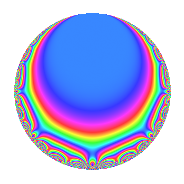# Properties

 Label 804.2.a.eLevel 804 Weight 2 Character orbit 804.a Self dual Yes Analytic conductor 6.420 Analytic rank 0 Dimension 3 CM No Inner twists 1

# Related objects

## Newspace parameters

 Level: $$N$$ = $$804 = 2^{2} \cdot 3 \cdot 67$$ Weight: $$k$$ = $$2$$ Character orbit: $$[\chi]$$ = 804.a (trivial)

## Newform invariants

 Self dual: Yes Analytic conductor: $$6.41997232251$$ Analytic rank: $$0$$ Dimension: $$3$$ Coefficient field: 3.3.1076.1 Coefficient ring: $$\Z[a_1, \ldots, a_{5}]$$ Coefficient ring index: $$1$$ Fricke sign: $$-1$$ Sato-Tate group: $\mathrm{SU}(2)$

## $q$-expansion

Coefficients of the $$q$$-expansion are expressed in terms of a basis $$1,\beta_1,\beta_2$$ for the coefficient ring described below. We also show the integral $$q$$-expansion of the trace form.

 $$f(q)$$ $$=$$ $$q - q^{3} + ( -1 - \beta_{1} ) q^{5} + ( -2 - \beta_{1} + \beta_{2} ) q^{7} + q^{9} +O(q^{10})$$ $$q - q^{3} + ( -1 - \beta_{1} ) q^{5} + ( -2 - \beta_{1} + \beta_{2} ) q^{7} + q^{9} + ( 1 + \beta_{1} + \beta_{2} ) q^{11} + ( 1 - \beta_{1} - \beta_{2} ) q^{13} + ( 1 + \beta_{1} ) q^{15} + ( 1 - \beta_{1} - \beta_{2} ) q^{17} + ( -1 + \beta_{1} - \beta_{2} ) q^{19} + ( 2 + \beta_{1} - \beta_{2} ) q^{21} + ( 3 - \beta_{1} ) q^{23} + ( 1 + 3 \beta_{1} + \beta_{2} ) q^{25} - q^{27} + 8 q^{29} + ( 1 - 2 \beta_{2} ) q^{31} + ( -1 - \beta_{1} - \beta_{2} ) q^{33} + ( 6 + 2 \beta_{1} + \beta_{2} ) q^{35} + ( 6 - \beta_{1} + \beta_{2} ) q^{37} + ( -1 + \beta_{1} + \beta_{2} ) q^{39} + ( 4 + 2 \beta_{1} + \beta_{2} ) q^{41} + ( -1 - 2 \beta_{1} + 2 \beta_{2} ) q^{43} + ( -1 - \beta_{1} ) q^{45} + ( 1 + 3 \beta_{1} - \beta_{2} ) q^{47} + ( 8 - 2 \beta_{2} ) q^{49} + ( -1 + \beta_{1} + \beta_{2} ) q^{51} + 3 \beta_{2} q^{53} + ( -7 - 5 \beta_{1} - \beta_{2} ) q^{55} + ( 1 - \beta_{1} + \beta_{2} ) q^{57} + ( 4 - 3 \beta_{2} ) q^{59} + ( -1 + \beta_{1} + 3 \beta_{2} ) q^{61} + ( -2 - \beta_{1} + \beta_{2} ) q^{63} + ( 5 + 3 \beta_{1} + \beta_{2} ) q^{65} - q^{67} + ( -3 + \beta_{1} ) q^{69} + ( 5 - 5 \beta_{1} - \beta_{2} ) q^{71} + ( -7 - 2 \beta_{1} - 2 \beta_{2} ) q^{73} + ( -1 - 3 \beta_{1} - \beta_{2} ) q^{75} + ( 1 - 5 \beta_{1} - 3 \beta_{2} ) q^{77} + ( -6 - 2 \beta_{2} ) q^{79} + q^{81} + ( 6 - 3 \beta_{2} ) q^{83} + ( 5 + 3 \beta_{1} + \beta_{2} ) q^{85} -8 q^{87} + ( 3 + \beta_{1} + 3 \beta_{2} ) q^{89} + ( -5 + 3 \beta_{1} + 5 \beta_{2} ) q^{91} + ( -1 + 2 \beta_{2} ) q^{93} + ( -3 + \beta_{1} - \beta_{2} ) q^{95} + ( 5 + \beta_{1} - \beta_{2} ) q^{97} + ( 1 + \beta_{1} + \beta_{2} ) q^{99} +O(q^{100})$$ $$\operatorname{Tr}(f)(q)$$ $$=$$ $$3q - 3q^{3} - 3q^{5} - 5q^{7} + 3q^{9} + O(q^{10})$$ $$3q - 3q^{3} - 3q^{5} - 5q^{7} + 3q^{9} + 4q^{11} + 2q^{13} + 3q^{15} + 2q^{17} - 4q^{19} + 5q^{21} + 9q^{23} + 4q^{25} - 3q^{27} + 24q^{29} + q^{31} - 4q^{33} + 19q^{35} + 19q^{37} - 2q^{39} + 13q^{41} - q^{43} - 3q^{45} + 2q^{47} + 22q^{49} - 2q^{51} + 3q^{53} - 22q^{55} + 4q^{57} + 9q^{59} - 5q^{63} + 16q^{65} - 3q^{67} - 9q^{69} + 14q^{71} - 23q^{73} - 4q^{75} - 20q^{79} + 3q^{81} + 15q^{83} + 16q^{85} - 24q^{87} + 12q^{89} - 10q^{91} - q^{93} - 10q^{95} + 14q^{97} + 4q^{99} + O(q^{100})$$

Basis of coefficient ring in terms of a root $$\nu$$ of $$x^{3} - 8 x - 6$$:

 $$\beta_{0}$$ $$=$$ $$1$$ $$\beta_{1}$$ $$=$$ $$\nu$$ $$\beta_{2}$$ $$=$$ $$\nu^{2} - \nu - 5$$
 $$1$$ $$=$$ $$\beta_0$$ $$\nu$$ $$=$$ $$\beta_{1}$$ $$\nu^{2}$$ $$=$$ $$\beta_{2} + \beta_{1} + 5$$

## Embeddings

For each embedding $$\iota_m$$ of the coefficient field, the values $$\iota_m(a_n)$$ are shown below.

For more information on an embedded modular form you can click on its label.

Label $$\iota_m(\nu)$$ $$a_{2}$$ $$a_{3}$$ $$a_{4}$$ $$a_{5}$$ $$a_{6}$$ $$a_{7}$$ $$a_{8}$$ $$a_{9}$$ $$a_{10}$$
1.1
 3.14743 −0.818558 −2.32887
0 −1.00000 0 −4.14743 0 −3.38854 0 1.00000 0
1.2 0 −1.00000 0 −0.181442 0 −4.69285 0 1.00000 0
1.3 0 −1.00000 0 1.32887 0 3.08139 0 1.00000 0
 $$n$$: e.g. 2-40 or 990-1000 Significant digits: Format: Complex embeddings Normalized embeddings Satake parameters Satake angles

## Inner twists

This newform does not admit any (nontrivial) inner twists.

## Atkin-Lehner signs

$$p$$ Sign
$$2$$ $$-1$$
$$3$$ $$1$$
$$67$$ $$1$$

## Hecke kernels

This newform can be constructed as the kernel of the linear operator $$T_{5}^{3} + 3 T_{5}^{2} - 5 T_{5} - 1$$ acting on $$S_{2}^{\mathrm{new}}(\Gamma_0(804))$$.